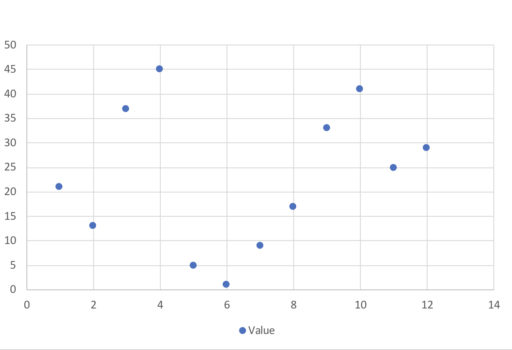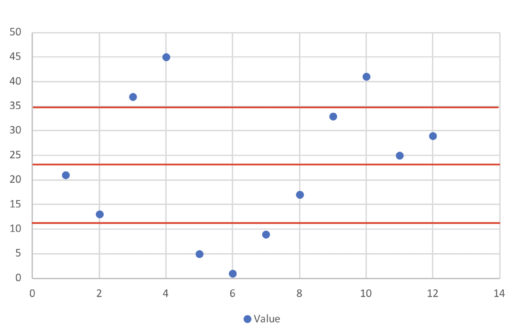In this post, we'll talk about ranges, quartiles, and percentiles. These are all ways of getting a sense of what the overall distribution looks like and what the possible outcomes look like.

Let's start with the range. The range is the difference between the largest and the smallest value in your data. So suppose you have the following data:Then, the range is. Thus, the range tells you the interval over which your data is distributed.

Quartiles are more complicated. As the name suggests, quartiles are a way of dividing up the data in to four parts. So, if we have the following data:The quartiles are the points that divide up the data in four segments, each with the same number of observations. For the above data, we would get:You can see how in each section, we have exactly three points which is one fourth of our total data (made up of 12 points).

More formally, the second quartile is the median. Then, the first quartile is the median of all the values that are less than the median (or second quartile). The third quartile is the median of all the values that are greater than the median.

Now how can we find the quartiles?

Well, we already know how to find the second quartile since that's just the median. After finding the median, separate the data into two halves: the lower half is made up of all the values smaller than our median, whereas the higher half is all the values greater than our median.

Then, find the median of both halves. The median of the lower half will be the first quartile, and the median of the upper half will be the second quartile value.

This makes some intuitive sense since 25% is exactly at the middle between 0 and 50%, which is the data that makes up our lower half, whereas 75% is the middle of 50% and 100%, which is the data that makes up our upper half. So if we were looking for a process to split the data into four parts, each containing 25% of our observations, this method (to find the quartiles) would be an excellent candidate for that task.

Finally, the interquartile range is just the value of the third quartile minus the value of the first quartile.

Example 1

Find the first, second, and third quartiles of: 21, 13, 37, 45, 5, 1, 9, 17, 33, 41, 25, 29. Then find the interquartile range.

Practice Problems

1. Find the first, second, and third quartiles of: 30, 22, 90, 43, 28, 65, 2, 12. Then, find the interquartile range. Also, find the range.
2. Find the first, second, and third quartiles for: 111, 210, 291, 240, 287, 534, 323, 222, 401. Then, find the interquartile range. Also, find the range.
3. For the following numbers, is the interquartile range larger than the range? 22, 12, 32, 53, 46, 23, 21, 19, 35, 42, 31, 21, 24